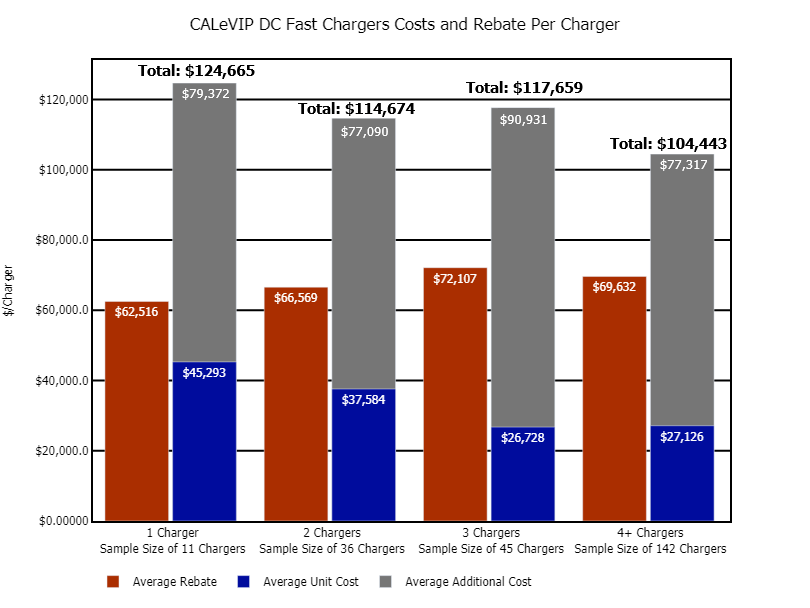The bar graph shows the average rebate per charger, the unit cost per charger, and the average total project cost per charger aggregated by the number of direct current (DC) fast chargers installed.

For 1 installed DC fast charger, the average rebate per charger was \$62,516, the average unit cost per charger was \$45,293, and the average total project cost per DC fast charger was \$124,665. For 2 installed DC fast chargers, the average rebate per DC fast charger was \$66,569, the average unit cost per charger was \$37,584, and the average total project cost per DC fast charger was \$114,674. For 3 installed DC fast chargers, the average rebate per DC fast charger was \$72,107, the average unit cost per charger was \$26,728, and the average total project cost per DC fast charger was \$117,659. For 4 or more installed DC fast chargers, the average rebate per DC fast charger was \$69,632, the average unit cost per charger was \$27,126, and the average total project cost per DC fast charger was \$104,443. This graph excludes sites that installed both Level 2 and DC fast chargers as a single project. This graph excludes some DC fast charger sites where the unit cost was undefined because of aggregated equipment costs.